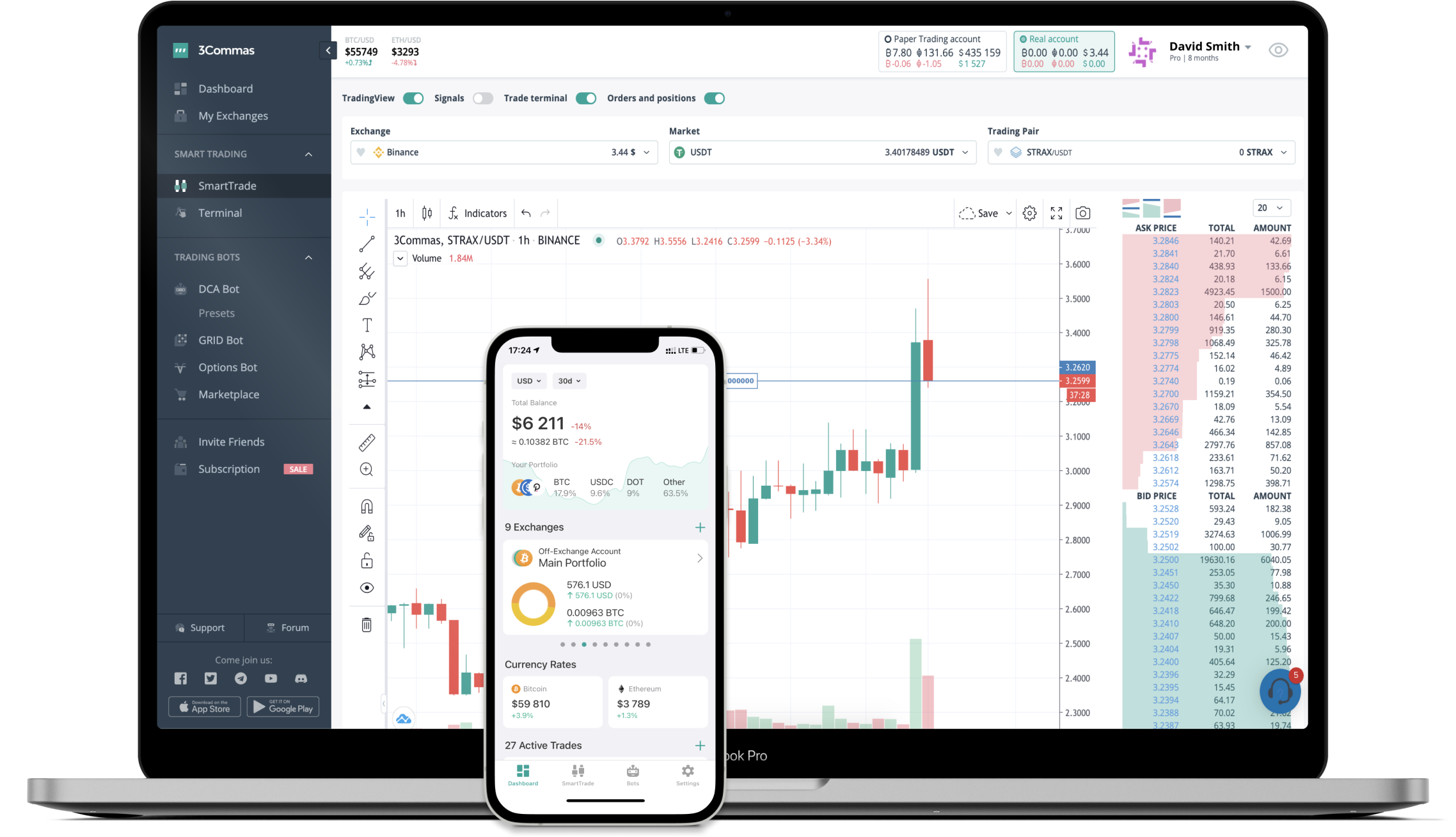# EQUAL to ETH Converter

Equalizer DEX
PriceETH 0.00398854-4.81%
VolumeETH 75
How it work

The 3Commas currency calculator allows you to convert a currency from Equalizer DEX (EQUAL) to Ethereum (ETH) in just a few clicks at live exchange rates.

Simply enter the amount of Equalizer DEX you wish to convert to ETH and the conversion amount automatically populates. You can also use our Prices Calculator Table to calculate how much your currency is worth in other denominations, i.e. .1 EQUAL, .5 EQUAL, 1 EQUAL, 5 EQUAL, or even 10 EQUAL.

## EQUAL to ETH Exchange Calculator

The chart displays Equalizer DEX price changes in ETH.

The ultimate tools for crypto traders to maximize trading profits while minimizing risk and loss.## Latest EQUAL to ETH price calculator

Equalizer DEX market price is updated every three minutes and is automatically displayed in ETH. Below are the most popular denominations to convert to ETH.

Equalizer DEX
Ethereum
0.00
ETH
0.00
ETH
0.00
ETH
0.01
ETH
0.01
ETH
0.02
ETH
0.04
ETH
0.10
ETH
0.20
ETH
0.40
ETH
1.00
ETH
1.99
ETH
3.99
ETH
9.97
ETH
Ethereum
Equalizer DEX
2.50718308
EQUAL
25.07183080
EQUAL
250.71830795
EQUAL
501.43661590
EQUAL
752.15492386
EQUAL
1253.59153976
EQUAL
2507.18307952
EQUAL
6267.95769881
EQUAL
12535.91539761
EQUAL
25071.83079523
EQUAL
62679.57698807
EQUAL
125359.15397614
EQUAL
250718.30795228
EQUAL
626795.76988071
EQUAL

## Top Currencies